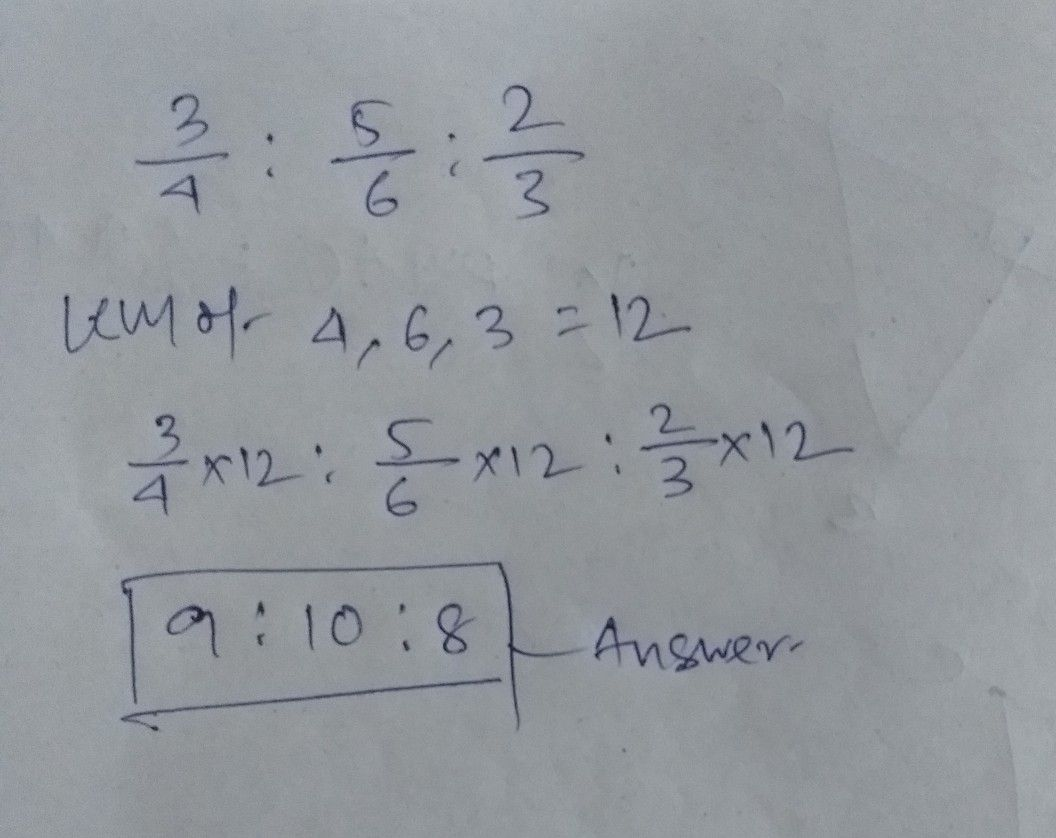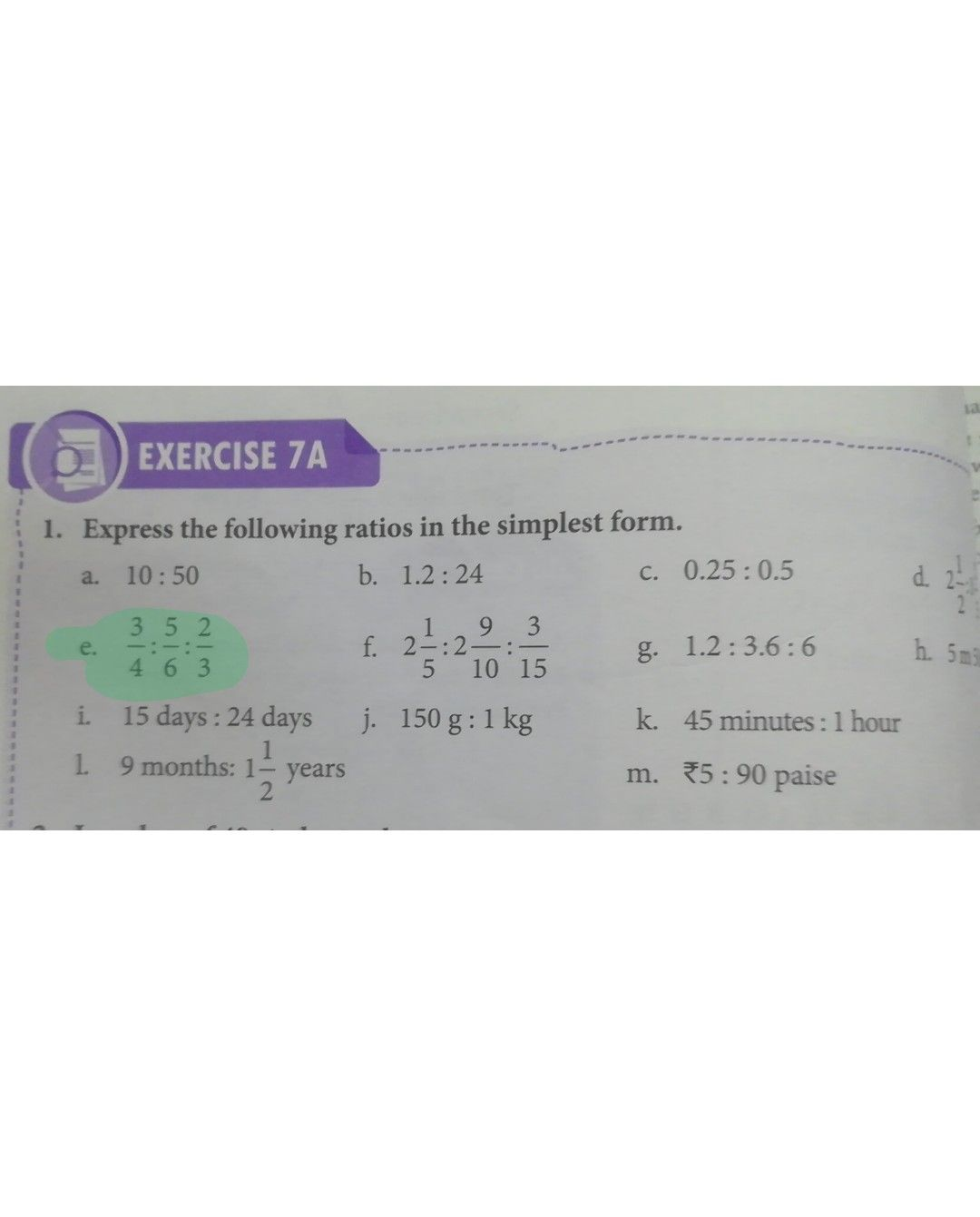Symbol
Problem$\dfrac {3} {4}:\dfrac {5} {6}:\dfrac {2} {3}$
7th-9th grade
Algebra
SolutionQanda teacher - AmibisuStudent
the answer is wrong please try it again
You need to express in ratio
the answer is 15:8Qanda teacher - Amibisu
there is ratio between three no
how the ans is like this
what is the actual questionStudent
in my book the answer is 15:8
And question is 3/4 : 5/6 : 2/3Qanda teacher - Amibisu
can you send me the instructions what is to findStudent
ok sir just waitis it??Qanda teacher - Amibisu
yes the solution is correct
the answer in your book may be wrongStudent
ok sir thank you soo much ☺️Qanda teacher - Amibisu
because you see clearly it is a ratio among 3 no how the answer is ratio between 2 noStudent
yes
thanks againQanda teacher - Amibisu
all the best dearStudent
thanks